## Tuesday, July 22, 2008

### BellKor Algorithm: Pearson Correlation Coefficient

Introduction

In their paper on the k-nearest neighbor (knn) algorithm, the BellKor team mention three choices that they considered for a similarity function: Pearson's Correlation Coefficient, the Cosine Coefficient, and the Root Mean Square Difference.

In today's blog, I will focus solely on the Pearson Correlation Coefficient. It is clear from the paper that they did a lot of work with Pearson. There is also a good explanation about it in the book Collective Intelligence.

In line with the BellKor article, I will focus on the item-item correlation. Newman! and Tillberg have reported that they can generate this statistic in 20 minutes on a single processor and others have reported generating it in 5 minutes on a quad-core processor. The algorithm that I describe in today's blog took 2 hours to run and required 1GB RAM on my machine. It look 45 minutes to load up into mysql. I wrote the algorithm in java. I suspect that a C/C++ algorithm can go faster and the code is not well optimized. My goal here is to present the algorithm in the clearest possible way.

MySQL Tables

It is assumed that a Rating table already exists in mysql that includes all the Netflix training data. In a previous blog, I went into detail on how I loaded this table from the Netflix data and removed the probe data. Here is the table:

Rating Table
• movieid: int(2)
• userid: int(3)
• rating: int(1)
• date: date
• first: date
• support: int(3)
• avg: double
• avg2: double
• residual: double
In addition to this table, I created a table which I called Pearson. Here is the sql for creating this table:
`CREATE TABLE pearson (movie1 int(2), movie2 int(2), num int(3), pearson float,index index_pearson_movie1(movie1),index index_pearson_pearson(pearson));`

So, we end up with the following table:

Pearson Table
• movie1: int(2)
• movie2: int(2)
• num: int(3)
• pearson: float
Now, the tricky part is generating the pearson correlation coefficient for all 17,770 tables. That's a set of 17,770 x 17,770 = over 315 million records. On a very powerful machine, that may not be a problem but one a 1GB machine, if you do not code the algorithm correctly, the processing can easily take more than 2 hours.

But before jumping into the algorithm, let's take a look at the Pearson formula. One of the important optimizations comes down to simplifying the standard formula.

Pearson Formula

Here's the formula for computing the coefficient for two items x and y is the following: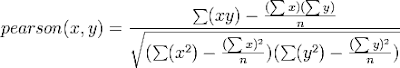Following the cue from the book Collective Intelligence by O'Reilly, it gets much simpler, if we decompose it into the following 5 variables: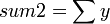Then, the formula becomes:The result will be between -1 and 1 where the closer it is to 1, the closer the match. A movie compared to itself will score a 1.

Loading up the Ratings table into memory

In order to calculate the pearson coefficient quickly, we need to load up the Rating table into memory and we need to have it sorted by both movieid and userid.

Here is what Dan Tillberg writes in the Netflix Prize forum:
...the trick is to have trainer ratings sorted by both movie and by user. Then, to find movie-movie similarities for, say, movie m1, you look at all of the *users* that rated movie m1, and then you go through each of those users' *movies* that they've rated to fill in the entire m1'th row of the movie-movie similarity matrix (actually, you only need to bother with movies m2 <= m1, since the other half is symmetrical, as noted further below). I do this in three minutes on an quad-core processor. If you calculate it cell by cell instead of row by row it takes a lot longer.
I'll talk about the computation of the coefficient in the next section. On my 1GB machine, I needed to run java with the following options:

`java -Xms950m -Xmx950m pearson > pearson.txt`

So, the goal is to output the result to a file pearson.txt which I will later load up into MySQL.

To speed things up, I don't fully sort the ratings by userid and the movieid. Instead, I group all the ratings by userid and then I group all the ratings by movieid. This makes the load up into memory go significantly faster.

Here is the arrays that I used for the load up:

`private static final int NUM_RECORDS=99072112;byte[] ratingByUser = new byte[NUM_RECORDS];short[] movieByUser = new short[NUM_RECORDS];byte[] ratingByMovie = new byte[NUM_RECORDS];int[] userByMovie = new int[NUM_RECORDS];`

To group each rating, I use the following arrays:

`private static final int NUM_USERS=480189;private static final int NUM_MOVIES=17770;int[] userIndex = new int[NUM_USERS+1];int[] userNextPlace = new int[NUM_USERS];int[] movieIndex = new int[NUM_MOVIES+1];int[] movieNextPlace = new int[NUM_MOVIES];`

Some Help from MySQL

To speed up the processing, I create two tables in MySQL that will provide me with the number of movies by user and the number of users by movie. These will be relatively small tables and that will help in the grouping process. I will use the term "support" in the same way BellKor where "movie support" is the number of users who rated each movie and "user support" is the number of movies rated each user.

Here are the two tables:

Movie_Support
• movieid: int(2)
• support: int(3)
User_Support
• userid: int(3)
• support: int(3)
Here's the sql for creating and loading up these tables:

`CREATE TABLE movie_support (movieid INT(2), support INT(3));CREATE TABLE user_support (userid INT(3), support INT(3));INSERT INTO movie_support (movieid,support) SELECT movieid, COUNT(rating) FROM rating GROUP BY movieid;INSERT INTO user_support (userid,support) SELECT userid, COUNT(rating) FROM rating GROUP BY userid;`

We don't need any indexes for either of these tables.

Handling UserId

UserId presents an opportunity for reducing storage space. Now, the userid data presented as part of training varies from 6 to 2,649,429. But, there are only 480,189 distinct users.

To address this point, I use a simple hash table to map a relative userid (1 .. 480,189) to the absolute userid (6 .. 2,649,429). Here's the code to do this:

`private static final int nextRelId=0;private static HashMap<integer,integer> userMap = new HashMap<integer,integer>();private static int getRelUserId(int userId) {Integer relUserId = userMap.get(userId);if (relUserId == null) {userMap.put(userId,nextRelUserId);relUserId = nextRelId++;}return relUserId;}`

Before Grouping Ratings by Movie and User

To prepare for the load up all the ratings and group them by userid and movieid, I used the following java code:

`userIndex[NUM_USERS] = NUM_RECORDS;int i=0;ResultSet rs = stmt.executeQuery("SELECT userid,support FROM user_support");while (rs.next()) {int relUserId = getRelUserId(rs.getInt("userid"));userIndex[relUserId] = i;userNextPlace[relUserId] = i;i += rs.getInt("support");}rs.close();movieIndex[NUM_MOVIES] = NUM_RECORDS;i = 0;rs = stmt.executeQuery("SELECT movied,support FROM movie_support");while (rs.next()) {int relMovieId = rs.getInt("movieid")-1;movieIndex[relMovieId]=i;movieNextPlace[relMovieId]=i;i += rs.getInt("support");}rs.close();`

Once, I have these indices set, I am ready to start loading up. The advantage of these arrays will now become apparent. We do a very fast single pass through the entire Rating table.

Grouping the Ratings by User and Movie

Here's the java code for loading up the ratings:

`rs = stmt.executeQuery("SELECT userid,movieid,rating FROM rating");while (rs.next()) {int userId = rs.getInt("userid");int relUserId = getRelUserId(userid);short movieId = rs.getShort("movieid");int relMovieId = movieId-1;byte rating = rs.getByte("rating");movieByUser[userNextPlace[relUserId]] = movieId;ratingByUser[userNextPlace[relUserId]] = rating;userNextPlace[relUserId]++;userByMovie[movieNextPlace[relMovieId]] = userId;ratingByMovie[movieNextPlace[relMovieId]] = rating;movieNextPlace[relMovieId]++;}rs.close();`

Quickly Tallying the Ratings

Once we've loaded up ratings into memory, we are ready to process them. To do this efficiently, I use a technique that Newman! posted on the Netflix Prize forum. Here's Newman's description:

If you're using raw score values (1 to 5), you can speed things up significantly by replacing the PearsonIntermediate structure with:

unsigned int32 viewerCnt;

where viewerCnt[m][n] is the number of viewers who gave movie X a score of m+1 and movie Y a score of n+1. Furthermore, based on the total number of viewers of a movie, you can replace int32 with int16 or int8, speeding it up even further.

On my 1.5G laptop, it took 20 minutes to calculate 17770x17770 correlations and write out a big data file. Of course, you really need to calculate only 17770x17770/2, if you have enough memory to hold all correlation and related values (like average rating from common viewers) in memory, otherwise disk seeking will kill your running time and hard disk when you read the data file later. On a multi-core processor, you can easily parallelize the calculations. So I think on a fast multi-core system, you can calculate all movie correlations in less than 5 minutes.

So, here's my code:
`int[][][] values = new int[NUM_MOVIES];for (i=0; i < NUM_MOVIES-1; i++) {for (int j=i+1; j < NUM_MOVIES; j++) {for (int k=0; k < 5; k++) {for (int l=0; l < 5; l++) {values[j][k][l]=0;}}}for (int j=movieIndex[i]; j < movieIndex[i+1]; j++) {int relUserId = getRelUserId(userByMovie[j]);for (int k=userIndex[relUserId]; k < userIndex[relUserId+1]; j++) {if (movieByUser[k]-1 > i) {values[movieByUser[k]-1][ratingByUser[k]-1][ratingByMovie[j]-1]++;}}}}`
Shrinking the Pearson Coefficient in relation to data available

BellKor state that they shrink the pearson coefficient based on the the count of data available. They use the following formula in the article: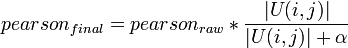Where |U(i,j)| is "the set of users hwo rated both items j and k".

In the original article, they state that they used "some small α." In the NetPrize Forum, Yehuda Koren thinks that they "used alpha values around 5-10." In my code, I use α = 10.

Computing the Pearson

With this tally, I am now able to compute the Pearson coefficient for each movie in comparison to i.

Here's the code:

`for (i=0; i < NUM_MOVIES-1; i++) {// Insert the code from above for tallying the totals...//...for (int j=i+1; j < NUM_MOVIES; j++) {float sum1=0;float sum2=0;float sumsq1=0;float sumsq2=0;float sumpr=0;float num=0;for (int k=1; k <= 5; k++) {for (int l=1; k <= 5; l++) {int val=values[j][k-1][l-1];sum1 += l*val;sum2 += k*val;sumsq1 = l*l*val;sumsq2 = k*k*val;sumpr = k*l*val;num += val;}}float top = sumpr - (sum1*sum2/num);float bottom = (float)Math.sqrt((sumsq1 - (sum1*sum1)/num)*(sumsq2 - (sum2*sum2)/num));if (bottom != 0) {float pearson = (top/bottom)*(num/(num+10));System.out.print((i+1)+","+(j+1)+","+num+","+pearson+"\n");System.out.print((j+1)+","+(i+1)+","+num+","+pearson+"\n");}else {System.out.print((i+1)+","+(j+1)+","+num+","+0+"\n");}}}`

Loading Pearson Coefficients into MySQL

Here's the sql that I used to load back up into MySQL

`use netflix;ALTER TABLE pearson DISABLE KEYS;LOAD DATA LOCAL INFILE 'pearson.txt' INTO TABLE pearsonFIELDS TERMINATED BY ','LINES TERMINATED BY '\n'(movie1,movie2,num,pearson);ALTER TABLE pearson ENABLE KEYS;`

That's pretty much it.

Using Your Pearson Table

For example to compute 10 movies that are most like The Adventures of Robin Hood (movieid 16840), we do the following:
`SELECT movie1,pearson FROM pearson WHERE movie2=7064 ORDER BY pearson DESC LIMIT 10;`

Now, I ran my pearson against the entire training data so your results may be different.

Here are my results:

References

## Tuesday, May 27, 2008

### BellKor Algorithm: Global Effects

Introduction

This article refers to the BellKor Algorithm which won the \$50,000 Progress Prize as part of the Netflix Prize contest. The purpose of this article is to provide details on the winning algorithm.

The BellKor team found that removing Global Effects greatly improved the results of applying the K Nearest Neighbor (knn) Algorithm.

In today's blog, I will use MySQL to remove Global Effects in the effort to reproduce the results of the BellKor paper.

Disclaimer

I am not an expert in learning theory or statistics. The work done here is my best effort with some support from the Netflix Prize forum. While I was able to reproduce each of the effects that the BellKor identified, I did not get an exact match on some of the numbers. This indicates that the details provided here may not be exactly the same as done by BellKor.

I am very glad to be corrected by anyone who is able to get an exact match for the BellKor results or is an expert of the field.

MySQL

I chose MySQL because my goal here is to understand the logic while minimizing the amount of coding involved. While using memory mapped files with C/C++ is probably the fastest way to process data on Windows, I found that MySQL with indexed tables and occasional help from java/jdbc was fine for me.

For those interested in the steps I followed to load up the Netflix Prize Data into MySQL and remove the probe data, you can see the details here. The set up described there took me roughly 3 hours to complete.

If anyone has suggestions for reducing the time it takes or improving the calculations, please feel free to post it here.

After following this process, I have the following two tables:

Rating Table
• movieid: int(2)
• userid: int(3)
• rating: int(1)
• date: date
• first: date
• support: int(3)
• avg: double
• avg2: double
• residual: double
The rating table has 99,072,112 ratings which do not include the probe table data.

Probe Table
• movieid: int(2)
• userid: int(3)
• rating: int(1)
• date: date
• first: date
• support: int(3)
• avg: double
• avg2: double
• prediction: double
The probe table has 1,408,395 ratings.

Global Effects

Some users are easier on movies when they select their rating. If they like or hate a movie, they rate it a bit higher. Some uses are tougher in their ratings and they rate movies just a little bit lower. Both of these tendencies are examples of a "global effect." In this case, what the BellKor team call a "user effect". Some movies get higher ratings when they are first released than after they've been out for a while. This is an example of a "movie x time (movie) effect."

In their paper, the BellKor team present a strategy of removing a global effect "one 'effect' at a time." In this way, it is possible to test for "effects", identify them, and then remove them. After each removal, the RMSE goes down (see here for explanation about RMSE if needed).

The idea of "global effects" is very enticing. You identify an effect, you test for it, and then you remove it for the total. As long as you keep finding effects, in theory, the closer you get to the goal of an RMSE=0.85. In practice, the BellKor team found that global effects alone only got them to an RMSE=0.96. In this paper, I will go through each of the 11 global effects that the BellKor team identified in their paper.

Residuals

Since the goal is to remove each "effect" one at a time, the plan is not to focus on the ratings themselves but rather to focus on the "residuals" which are the ratings minus an effect.

To make this point as clear as possible, I will refer to rui as the rating of user u on item i. I will refer to each residual as rui(n) where n refers to the number of effects that have been removed.

For example:

rui(0) = rui.

rui(1) = rui(0) - effect1.

rui(2) = rui(1) - effect2

and so on until we have:

rui(n) = rui(n-1) - effectn.

The model for the effect is:

effectn = θ*xui

where:

xui = variable of interest

and

θ = effectn/xui

Variable of Interest

BellKor distinguishes between three types of effects:

(1) Main Effects

In this case, xui = 1

(2) User Effects

In this case, xui = xui - avg(xui for a given u)

(3) Item Effects

In this case, xui = xui - avg(xui for a given i)

They succinctly call this out by writing:
For other global effects, we center xui for each user by subtracting the mean of xui for that user.
Estimating θ

To estimate θ, the BellKor paper starts by looking at the idea of an unbiased estimator.

An estimator is a function on sample variables that "estimates" a population variable. An "unbiased estimator" is one where if the sample size is large enough, the estimator is equal to the population variable estimated.

BellKor proposes the following function for estimating the "effect" using an unbiased estimator: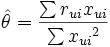Note: you can tell we are using an "estimator" because of the "hat" atop θ.

The problem with this approach is that in many cases, the sample size is not very large for a given user and there is always the chance that the sample is not large enough for a given item.

Using a Posterior Mean

To compensate for this, BellKor use the concept of a posterior mean. This can be derived by making assumptions about the normal distribution.

If we assume that for θ, the population mean is μ and the population variance is τ2, then θ can be characterized:
θ ∼ N(μ,τ2)

Further, using the sample variance σ2, the BellKor paper notes: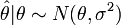Using these two observations, they now restate the estimator for θ as its posterior mean:Assumptions on μ and σ2

Now, since all parameters are centered (that is, x = x - avg(x)), we can assume that the population mean is 0. That is μ = 0.

BellKor further assume that σ2 is proportional ot 1/count(rui). If we assume that n = count(rui) then there exists a k such that σ2 = k/n.

Then, the revised formula is:BellKor now states a very complicated formula for estimating τ2:Ouch. Well, let's simplify it using the above assumptions. This gets us to:Better. But then, BellKor do a "magic" step which is not clear to me at this point. They say that
it is possible to greatly simplify the above analysis into the following formula:

effect =where α is a value calculated through cross-validation and n is the number of ratings.

Resulting Formula for Effect

So, after all the above analysis, the formula for effect is as follows:

effect =The List of Global Effects

In their paper, BellKor list the following "effects":
1. Overall Mean (RMSE = 1.1296)
2. Movie Effect (RMSE = 1.0527)
3. User Effect (RMSE = 0.9841)
4. User x Time (user)1/2 (RMSE = 0.9809)
5. User x Time (movie)1/2 (RMSE = 0.9786)
6. Movie x Time (movie)1/2 (RMSE = 0.9767)
7. Movie x Time (user)1/2 (RMSE = 0.9759)
8. User x Movie average (RMSE = 0.9719)
9. User x Movie support (RMSE = 0.9690)
10. Movie x User average (RMSE = 0.9670)
11. Movie x User support (RMSE = 0.9657)
First, I go into more detail about cross validation and the resulting α. Then, I will now proceed to show my effort to reproduce each effect using sql.

Cross-Validation Values

Yehuda Koren of BellKor has provided the alpha values that they used:
Movie: 25, 1.0527
User: 7, 0.9841
User-time(user): 550, 0.9809
User-time(movie): 150, 0.9786
Movie-time(movie): 4000, 0.9767
Movie-time(user): 500, 0.9759
User-mean(movie): 90, 0.9719
User-support(movie): 90, 0.9690
Movie-mean(user): 50, 0.9670
Movie-support(user): 50, 0.9658
In general, cross-validation is done simply by changing values. For example, if you use user_effect and set α = 6 and then α = 8, you will see the following pattern:

User effect: α = 6, RMSE = 0.984506
User effect: α = 7, RMSE =0.984436
User effect: α = 8, RMSE = 0.984434
User effect: α = 9, RMSE = 0.984485

Overall Mean

The formulas for the over all mean are:Using sql, we have:

`select sum(rating)/count(rating) from rating;`

After less than a minute, the result is 3.6033.

To test out rmse for the overall mean, I run:

`update probe set prediction = 3.6033;create view rmse_probe as select sqrt(sum((rating - prediction)*(rating - prediction))/count(rating)) "rmse" from probe;select * from rmse_probe;`

From this select, I get RMSE = 1.1296. That's a match to the BellKor results.

This then gives us:

rui(1) = rui - [overall mean]

To update, the rating table, we do the following:

`update rating set residual = rating - 3.6033;`

This took almost 9 minutes on my machine.

Movie Effect

The formula here is:

xui = 1movie effect = θi*xui = θi*1 = θi

The SQL for this is:

`create table movie_effect (movieid int(2), sum_r_ui_x_ui double, sum_x_ui_squared double, n_i int(3), theta_i_hat double, theta_i double);insert into movie_effect (movieid, sum_r_ui_x_ui, sum_x_ui_squared, n_i) select movieid, sum(residual), count(residual), count(residual) from rating group by movieid;update movie_effect set theta_i_hat = sum_r_ui_x_ui/sum_x_ui_squared;update movie_effect set theta_i = (n_i*theta_i_hat)/(n_i + 25);create index index_movie_effect_movieid on movie_effect(movieid);`

On my machine, it completed in roughly 3 minutes.

To test it on probe, you do the following:

`alter table movie_effect engine=memory;update probe p set prediction = prediction + (select theta_i from movie_effect where movieid = p.movieid);`

The RMSE that I get here is: 1.0527. That's a match to the BellKor results.

rui(2) = rui(1) - [movie effect]

To update, the rating table, we do the following:

`update rating r set residual = residual - (select theta_i from movie_effect where movieid=r.movieid);alter table movie_effect engine=myisam;`

User Effect

The formula here is:

xui = 1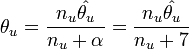user effect = θu*xui = θu*1 = θu

Here's the SQL (note: on my system, the user_effect table is too big for memory, so I created a special table memory_user_effect):

`create table user_effect (userid int(3), sum_r_ui_x_ui double, sum_x_ui_squared double, n_u int(3), theta_u_hat double, theta_u double);insert into user_effect (userid, sum_r_ui_x_ui,sum_x_ui_squared,n_u) select userid, sum(residual), count(residual),count(residual) from rating group by userid;update user_effect set theta_u_hat = sum_r_ui_x_ui/sum_x_ui_squared;update user_effect set theta_u = (n_u*theta_u_hat)/(n_u + 7);create index index_user_effect_userid on user_effect(userid);set max_heap_table_size=32000000;alter table user_effect engine=memory;update probe p set prediction = prediction + (select theta_u from user_effect where userid=p.userid);update probe set prediction = 5 where prediction > 5;update probe set prediction = 1 where prediction < 1;`

The RMSE I get here is: 0.98404. That's a match to the BellKor results.

rui(3) = rui(2) - [user effect]

To update, the rating table, we do the following:

`update rating r set residual = residual - (select theta_u from user_effect where userid=r.userid);alter table user_effect engine=myisam;`

User x Time (user)1/2

The formula here is:

xui = √days - avg(√days)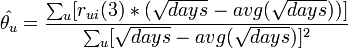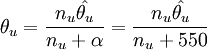user x time (user) effect = θu*[days - avg(√days)]

where days = sqrt(numOfDaysBetween(date,min(date) for user))

Here's what I did for the sql:

(1) Populate the "first" date field of tables: rating and probe

`create table user_first_rating as select userid,min(date) "first" from rating group by userid;create index index_user_first_rating_userid on user_first_rating(userid);alter table user_first_rating engine=memory;update rating r set first = (select first from user_first_rating where userid = r.userid);update probe p set first = (select first from user_first_rating where userid = p.userid);update probe p set first = date where datediff(date,first) < 0;`

(2) Populate "avg" field of tables: rating and probe

`create table user_avg_num_days_for_user (userid int(3), avg double);insert into user_avg_num_days_for_user (userid,avg) select userid,avg(sqrt(datediff(date,first))) from rating group by userid;create index index_user_avg_num_days_for_user_userid on user_avg_num_days_for_user(userid);alter table user_avg_num_days_for_user engine=memory;update rating r set avg = (select avg from user_avg_num_days_for_user where userid = r.userid);update probe p set avg = (select avg from user_avg_num_days_for_user where userid = p.userid);alter table user_avg_num_days_for_user engine=myisam;`

(3) Compute user_time_user_effect

`create table user_time_user_effect (userid int(3), sum_r_ui_x_ui double, sum_x_ui_squared double, n_u int(3), theta_u_hat double, theta_u double);insert into user_time_user_effect (userid,sum_r_ui_x_ui,sum_x_ui_squared,n_u) select userid,sum(residual*(sqrt(datediff(date,first)) - avg)),sum((sqrt(datediff(date,first)) - avg)*(sqrt(datediff(date,first))-avg)),count(residual) from rating group by userid;update user_time_user_effect set theta_u_hat = sum_r_ui_x_ui/sum_x_ui_squared;update user_time_user_effect set theta_u = (n_u*theta_u_hat)/(n_u + 550);update user_time_user_effect set theta_u = 0 where theta_u is null;create index index_user_time_user_effect on user_time_user_effect(userid);alter table user_time_user_effect engine=memory;update probe p set prediction = prediction + (select theta_u*(sqrt(datediff(date,first)) - avg) from user_time_user_effect where userid=p.userid);update probe set prediction = 5 where prediction > 5;update probe set prediction = 1 where prediction < 1;`

The RMSE I get here is: 0.9809. That's a match to the BellKor results.

rui(4) = rui(3) - [user x time(user) effect]

To update, the rating table, we do the following:

`update rating r set residual = residual - (select theta_u*(sqrt(datediff(date,first)) - avg) from user_time_user_effect where userid=r.userid);alter table user_time_user_effect engine=myisam;`

User x Time (movie)1/2

The formula here is:

xui = √days - avg(√days)user x time (user) effect = θu*[days - avg(√days)]

where days = sqrt(numOfDaysBetween(date,min(date) for movie))

Here's the sql:

(1) Populate the "first" date field of tables: rating and probe

`create table movie_first_rating as select movieid,min(date) "first" from rating group by movieid;create index index_movie_first_rating_movieid on movie_first_rating(movieid);alter table movie_first_rating engine=memory;update rating r set first = (select first from movie_first_rating where movieid = r.movieid);update probe p set first = (select first from movie_first_rating where movieid = p.movieid);alter table movie_first_rating engine=myisam;update probe p set first = date where datediff(date,first) < 0;`

(2) Populate the "avg" field of rating and probe

`create table user_avg_num_days_for_movie (userid int(3), avg double);insert into user_avg_num_days_for_movie (userid,avg) select userid,avg(sqrt(datediff(date,first))) from rating group by userid;create index index_user_avg_num_days_for_movie on user_avg_num_days_for_movie(userid);alter table user_avg_num_days_for_movie engine=memory;update rating r set avg = (select avg from user_avg_num_days_for_movie where userid = r.userid);update probe p set avg = (select avg from user_avg_num_days_for_movie where userid=p.userid);alter table user_avg_num_days_for_movie engine=myisam;`

(3) Compute user_time_movie_effect

`create table user_time_movie_effect (userid int(3), sum_r_ui_x_ui double, sum_x_ui_squared double, n_u int(3), theta_u_hat double, theta_u double);insert into user_time_movie_effect (userid,sum_r_ui_x_ui,sum_x_ui_squared,n_u) select userid,sum(residual*(sqrt(datediff(date,first)) - avg)),sum((sqrt(datediff(date,first)) - avg)*(sqrt(datediff(date,first))-avg)),count(residual) from rating group by userid;update user_time_movie_effect set theta_u_hat = sum_r_ui_x_ui/sum_x_ui_squared;update user_time_movie_effect set theta_u = (n_u*theta_u_hat)/(n_u + 150);update user_time_movie_effect set theta_u = 0 where theta_u is null;create index index_user_time_movie_effect on user_time_movie_effect(userid);alter table user_time_movie_effect engine=memory;update probe p set prediction = prediction + (select theta_u*(sqrt(datediff(date,first)) - avg) from user_time_movie_effect where userid=p.userid);update probe set prediction = 5 where prediction > 5;update probe set prediction = 1 where prediction < 1;`

The RMSE I get here is: 0.9785. That's a match to the BellKor results.

rui(5) = rui(4) - [user x time(movie) effect]

To update, the rating table, we do the following:

`update rating r set residual = residual - (select theta_u*(sqrt(datediff(date,first)) - avg) from user_time_movie_effect where userid=r.userid);alter table user_time_movie_effect engine=myisam;`

Movie x Time (movie)1/2

The formula here is: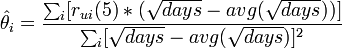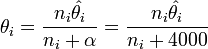where days = sqrt(numOfDaysBetween(date,min(date) for movie))

Here's the sql:

(1) Populate the "first" date field of tables: rating and probe

`alter table movie_first_rating engine=memory;update rating r set first = (select first from movie_first_rating where movieid = r.movieid);update probe p set first = (select first from movie_first_rating where movieid = p.movieid);update probe set first = date where datediff(date,first) < 0;alter table movie_first_rating engine=myisam;`

(2) Populate the "avg" field of rating and probe

`create table movie_avg_num_days_for_movie (movieid int(2), avg double);insert into movie_avg_num_days_for_movie (movieid,avg) select movieid,avg(sqrt(datediff(date,first))) from rating group by movieid;create index index_movie_avg_num_days_for_movie on movie_avg_num_days_for_movie(movieid);alter table movie_avg_num_days_for_movie engine=memory;update rating r set avg = (select avg from movie_avg_num_days_for_movie where movieid = r.movieid);update probe p set avg = (select avg from movie_avg_num_days_for_movie where movieid=p.movieid);alter table movie_avg_num_days_for_movie engine=myisam;`

(3) Compute movie_time_movie_effect

`create table movie_time_movie_effect (movieid int(2), sum_r_ui_x_ui double, sum_x_ui_squared double, n_i int(3), theta_i_hat double, theta_i double);insert into movie_time_movie_effect (movieid,sum_r_ui_x_ui,sum_x_ui_squared,n_i) select movieid,sum(residual*(sqrt(datediff(date,first)) - avg)),sum((sqrt(datediff(date,first)) - avg)*(sqrt(datediff(date,first))-avg)),count(residual) from rating group by movieid;update movie_time_movie_effect set theta_i_hat = sum_r_ui_x_ui/sum_x_ui_squared;update movie_time_movie_effect set theta_i = (n_i*theta_i_hat)/(n_i + 4000);update movie_time_movie_effect set theta_i = 0 where theta_i is null;create index index_movie_time_movie_effect on movie_time_movie_effect(movieid);alter table movie_time_movie_effect engine=memory;update probe p set prediction = prediction + (select theta_i*(sqrt(datediff(date,first)) - avg) from movie_time_movie_effect where movieid=p.movieid);update probe set prediction = 5 where prediction > 5;update probe set prediction = 1 where prediction < 1;`

The RMSE I get here is: 0.9766. That's a match to the BellKor results.

rui(6) = rui(5) - [movie x time(movie) effect]

To update, the rating table, we do the following:

`update rating r set residual = residual - (select theta_i*(sqrt(datediff(date,first)) - avg) from movie_time_movie_effect where movieid=r.movieid);alter table movie_time_movie_effect engine=myisam;`

Movie x Time (user)1/2

The formula here is: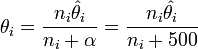wheredays = sqrt(numOfDaysBetween(date,min(date) for user))

Here's the sql:

(1) Populate the "first" date field of tables: rating and probe

`alter table user_first_rating engine=memory;update rating r set first = (select first from user_first_rating where userid = r.userid);update probe p set first = (select first from user_first_rating where userid = p.userid);update probe set first = date where datediff(date,first) < 0;`

(2) Populate "avg" field of tables: rating and probe.

`create table movie_avg_num_days_for_user (movieid int(2), avg double);insert into movie_avg_num_days_for_user (movieid,avg) select movieid,avg(sqrt(datediff(date,first))) from rating group by movieid;create index index_movie_avg_num_days_for_user_movieid on movie_avg_num_days_for_user(movieid);alter table movie_avg_num_days_for_user engine=memory;update rating r set avg = (select avg from movie_avg_num_days_for_user where movieid = r.movieid);update probe p set avg = (select avg from movie_avg_num_days_for_user where movieid = p.movieid);alter table movie_avg_num_days_for_user engine=myisam;`

(3) Compute movie_time_user_effect

`create table movie_time_user_effect (movieid int(2), sum_r_ui_x_ui double, sum_x_ui_squared double, n_i int(3), theta_i_hat double, theta_i double);insert into movie_time_user_effect (movieid,sum_r_ui_x_ui,sum_x_ui_squared,n_i) select movieid,sum(residual*(sqrt(datediff(date,first)) - avg)),sum((sqrt(datediff(date,first)) - avg)*(sqrt(datediff(date,first))-avg)),count(rating) from rating group by movieid;update movie_time_user_effect set theta_i_hat = sum_r_ui_x_ui/sum_x_ui_squared;update movie_time_user_effect set theta_i = (n_i*theta_i_hat)/(n_i + 500);create index index_movie_time_user_effect_movieid on movie_time_user_effect(movieid);alter table movie_time_user_effect engine=memory;update probe p set prediction = prediction + (select theta_i*(sqrt(datediff(date,first)) - avg) from movie_time_user_effect where movieid = p.movieid);update probe set prediction = 5 where prediction > 5;update probe set prediction = 1 where prediction < 1;`

The RMSE I get here is: 0.9758. That's a match to the BellKor results.

rui(7) = rui(6) - [movie x time(user) effect]

To update, the rating table, we do the following:

`update rating r set residual = residual - (select theta_i*(sqrt(datediff(date,first)) - avg) from movie_time_user_effect where movieid=r.movieid);alter table movie_time_user_effect engine=myisam;`

User x Movie average

The formula here is:Here's the sql:

(1) Populate the "avg" field of tables: rating and probe

`create table movie_average (movieid int(2), avg double);insert into movie_average (movieid,avg) select movieid,avg(rating) from rating group by movieid;create index index_movie_average_movieid on movie_average(movieid);alter table movie_average engine=memory;update rating r set avg = (select avg from movie_average where movieid = r.movieid);update probe p set avg = (select avg from movie_average where movieid = p.movieid);alter table movie_average engine=myisam;`

(2) Compute user_movie_average_effect

`create table user_movie_average_effect (userid int(3), sum_r_ui_x_ui double, sum_x_ui_squared double, n_u int(3), theta_u_hat double, theta_u double);insert into user_movie_average_effect (userid,sum_r_ui_x_ui,sum_x_ui_squared,n_u) select userid,sum(residual*(avg - 3.6033)),sum((avg - 3.6033)*(avg - 3.6033)),count(rating) from rating group by userid;update user_movie_average_effect set theta_u_hat = sum_r_ui_x_ui/sum_x_ui_squared;update user_movie_average_effect set theta_u = (n_u*theta_u_hat)/(n_u + 550);update user_movie_average_effect set theta_u = 0 where theta_u is null;create index user_movie_average_effect_userid on user_movie_average_effect(userid);alter table user_movie_average_effect engine=memory;update probe p set prediction = prediction + (select theta_u*(avg - 3.6033) from user_movie_average_effect where userid=p.userid);update probe set prediction = 5 where prediction > 5;update probe set prediction = 1 where prediction < 1;`

The RMSE I get here is: 0.9720. That's not an exact match but pretty close to the BellKor reported result of RMSE = 0.9719.

rui(8) = rui(7) - [user x movie average effect]

To update, the rating table, we do the following:

`update rating r set residual = residual - (select theta_u*(avg - 3.6033) from user_movie_average_effect where userid=r.userid);alter table user_movie_average_effect engine=myisam;`

User x Movie support

The formula here is:where support = the number of ratings for a given movie.

Here's the sql:

(1) Populate the "support" field of tables: rating and probe

`create table movie_support (movieid int(2), support int(3));insert into movie_support(movieid,support) select movieid,count(rating) from rating group by movieid;create index index_movie_support_movieid on movie_support(movieid);alter table movie_support engine=memory;update rating r set support = (select support from movie_support where movieid = r.movieid);update probe p set support = (select support from movie_support where movieid = p.movieid);alter table movie_support engine=myisam;`

(2) Populate avg field of tables: rating and probe

`create table average_movie_support_for_user (userid int(3), avg double);insert into average_movie_support_for_user(userid,avg) select userid,avg(sqrt(support)) from rating group by userid;create index index_average_movie_support_for_user_userid on average_movie_support_for_user(userid);alter table average_movie_support_for_user engine=memory;update rating r set avg = (select avg from average_movie_support_for_user where userid = r.userid);update probe p set avg = (select avg from average_movie_support_for_user where userid = p.userid);alter table average_movie_support_for_user engine=myisam;`

(3) Compute user_movie_support_effect

`create table user_movie_support_effect (userid int(3), sum_r_ui_x_ui double, sum_x_ui_squared double, n_u int(3), theta_u_hat double, theta_u double);insert into user_movie_support_ effect (userid,sum_r_ui_x_ui,sum_x_ui_squared,n_u) select userid,sum(residual*(sqrt(support) - avg),sum((sqrt(support) - avg)*(sqrt(support) - avg)),count(rating) from rating group by userid;update user_movie_support_effect set theta_u_hat = sum_r_ui_x_ui/sum_x_ui_squared;update user_movie_support_effect set theta_u = (n_u*theta_u_hat)/(n_u + 90);update user_movie_support_effect set theta_u = 0 where theta_u is null;create index index_user_movie_support_effect_userid on user_movie_support_effect(userid);alter table user_movie_support_effect engine=memory;update probe p set prediction = prediction + (select theta_u*(sqrt(support) - avg) from user_movie_support_effect where userid = p.userid);update probe set prediction = 5 where prediction > 5;update probe set prediction = 1 where prediction < 1;`

The RMSE I get here is: 0.9691. Still, extremely close to the BellKor reported result of RMSE = 0.9690.

rui(9) = rui(8) - [user x movie support effect]

To update, the rating table, we do the following:

`update rating r set residual = residual - (select theta_u*(sqrt(support) - avg) from user_movie_support_effect where userid=r.userid);alter table user_movie_support_effect engine=myisam;`

Movie x User average

The formula here is: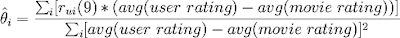Here's the sql:

(1) Populate the "avg" field of tables: rating and probe

`create table user_average (userid int(3), avg double);insert into user_average (userid,avg) select userid,avg(rating) from rating group by userid;create index index_user_average_userid on user_average(userid);alter table user_average engine=memory;update rating r set avg = (select avg from user_average where userid = r.userid);update probe p set avg = (select avg from user_average where userid = p.userid);alter table user_average engine=myisam;`

(2) Populate "avg2" field of tables: rating and probe

`alter table movie_average engine=memory;update rating r set avg2 = (select avg from movie_average where movieid = r.movieid);update probe p set avg2 = (select avg from movie_average where movieid = p.movieid);alter table movie_average engine=myisam;`

(3) Compute movie_user_average_effect

`create table movie_user_average_effect (movieid int(2), sum_r_ui_x_ui double, sum_x_ui_squared double, n_i int(3), theta_i_hat double, theta_i double);insert into movie_user_average_effect (movieid,sum_r_ui_x_ui,sum_x_ui_squared,n_i) select movieid,sum(residual*(avg - avg2),sum((avg - avg2)*(avg - avg2)),count(rating) from rating group by movieid;update movie_user_average_effect set theta_i_hat = sum_r_ui_x_ui/sum_x_ui_squared;update movie_user_average_effect set theta_i = (n_i*theta_i_hat)/(n_i + 50);create index index_movie_user_average_effect_movieid on movie_user_average_effect(movieid);alter table movie_user_average_effect engine=memory;update probe p set prediction = prediction + (select theta_i*(avg - avg2) from memory_movie_user_average_effect where movieid = p.movieid);update probe set prediction = 5 where prediction > 5;update probe set prediction = 1 where prediction < 1;`

The RMSE I get here is: 0.9680. While I caught an effect, my result is different than BellKor's reported result of RMSE = 0.9670.

rui(10) = rui(9) - [movie x user average effect]

To update, the rating table, we do the following:

`update rating r set residual = residual - (select theta_i*(avg - avg2) from movie_user_average_effect where movie=r.movieid);alter table movie_user_average_effect engine=myisam;`

Movie x User support

The formula here is: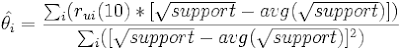where support = the number rating made by a given user.

Here is the sql:

(1) Populate the "support" field of tables: rating and probe

`create table user_support (userid int(3), support int(3));insert into user_support(userid,support) select userid,count(rating) from rating group by userid;create index index_user_support_userid on user_support(userid);alter table user_support engine=memory;update rating r set support = (select support from user_support where userid=r.userid);update probe p set support = (select support from user_support where userid=p.userid);alter table user_support engine=myisam;`

(2) Populate "avg" field of tables: rating and probe

`create table average_user_support_for_movie (movieid int(2), avg double);insert into average_user_support_for_movie(movieid,avg) select movieid,avg(support) from rating group by movieid;create index index_average_user_support_for_movie_movieid on average_user_support_for_movie(movieid);alter table average_user_support_for_movie engine=memory;update rating r set avg = (select avg from average_user_support_for_movie where movieid = r.movieid);update probe p set avg = (select avg from average_user_support_for_movie where movieid = p.movieid);alter table average_user_support_for_movie engine=myisam;`

(3) Compute movie_user_support_effect

`create table movie_user_support_effect (movieid int(2), sum_r_ui_x_ui double, sum_x_ui_squared double, n_i int(3), theta_i_hat double, theta_i double);insert into movie_user_support_effect (movieid,sum_r_ui_x_ui,sum_x_ui_squared,n_i) select movieid,sum(residual*(sqrt(support) - sqrt(avg))),sum((sqrt(support) - sqrt(avg))*(sqrt(support) - sqrt(avg))),count(rating) from rating group by movieid;update movie_user_support_effect set theta_i_hat = sum_r_ui_x_ui/sum_x_ui_squared;update movie_user_support_effect set theta_i = (n_i*theta_i_hat)/(n_i + 50);update movie_user_support_effect set theta_i = 0 where theta_i is null;create index movie_user_support_effect_movieid on movie_user_support_effect(movieid);alter table movie_user_support_effect engine=memory;update probe p set prediction = prediction + (select theta_i*(sqrt(support) - sqrt(avg) from movie_user_support_effect where movieid = p.movieid);update probe set prediction = 5 where prediction > 5;update probe set prediction = 1 where prediction < 1;`

The RMSE I get here is: 0.9658. That got me back on track to the BellKor reported result of RMSE = 0.9657.

rui(11) = rui(10) - [movie x user support effect]

To update, the rating table, we do the following:

`update rating r set residual = residual - (select theta_i*(avg - avg2) from movie_user_average_effect where movie=r.movieid);alter table movie_user_average_effect engine=myisam;`

Now, the trick is to find any other global effects to see how low you can go.

Observations from Netflix Forum

There is one particular thread on the NetflixPrize forum where the experts have spoken about global effects.

In this discussion, Gavin Potter (Just a Guy in a Garage ranked #4 at the time of the article) notes that he is able to get to an RMSE of 0.95 just using global effects. This impressed Yehuda Koren of the BellKor team who writes:

Gavin, I am very impressed by the RMSE=0.9503 achieved by pure global effects.

Yehuda Koren states that he does not believe that Global Effects alone will get someone to the NetFlix Prize. He writes:

Global effects are neat and intuitive, unlike the latent factor models (SVD and the likes) that by definition seek hidden, less explainable factors.
However, I don’t expect that improving global effects will have much impact on the final RMSE. Latent factor models were proven much more effective at recovering the same information and much beyond. Of course, I might be wrong about this...
In my next article, I will focus on the k-nearest neighbor (knn) algorithm and try to reproduce the BellKor results for global effects + knn.

Just to see what would happen, I ran the above "global effects" from the qualifying.txt and submitted to Netflix. The result against the qualifying data was an RMSE = 0.9665 which is very close to the RMSE = 0.9658 that I received against the probe data.

References

## Thursday, March 6, 2008

### BellKor Algorithm: Neighborhood Based Approach

The content that follows is taken from Robert M. Bell and Yehuda Koren's article "Improved Neighborhood-based Collaborative Filtering." This article is cited as the reference for the Neighborhood-based approach used in the BellKor algorithm.

The Neighborhood-based algorithm as described by the BellKor team has three major steps:

1) data normalization

In this step, an attempt is made to control for "global effects", that is, effects where certain users tend to rate movies higher or lower or it can also include interaction effects such that the probability that the Fellowship of the Rings, Two Towers, Return of the King will probably have similar ratings. Movies may get higher ratings when they first come out or lower ratings when they've been out a very long time. Some movies are have a large number of ratings. Some movies have a much smaller number.

For the probe data, predicting movie ratings based solely on the mean results in an RMSE of 1.1296 (see my earlier blog for review of RMSE if needed). Controlling for "global effects" gave this same date an RMSE of 0.9657 (according to the cited article).

In the actual kNN algorithm, residual data is processed. Using the algorithm without removing "global effects" resulted in an RMSE of 0.9536 (according to the cited article). Using kNN after removing "global effects" and matrix factorization data resulted in an RMSE of 0.9071 (according to the cited article).

I will not cover the removal of "global effects" or matrix factorization in today's blog. For purposes of focusing solely on the kNN algorithm, I wil assume that all data is raw (that is, it has not been normalized).

In a future blog, I will cover "global effects" and matrix factorization and try to reproduce the BellKor results cited.

2) neighbor selection

This is where a similarity function is selected and where this similiarity function is used to determine the k nearest neighbors. This can be approached in a user-oriented way (a function on similiar users) or in an item-oriented way (a function on simlar items).

I will go into detail about the formulas used in the article for the similarity function. In a future blog, I will get into code and compare the BellKor approach to other kNN approaches.

3) determination of the interpolation weights

Determination of interpolation weights is very important since they are tied very closed to the prediction. Bell and Koren compare results they reached with results from a "correlation-based interpolation."

They found that for raw scores, correlation-based interpolation resulted in an RMSE of 0.9947. In contrast, their approach resulted in an RMSE of 0.9536. After removing "global effects" and matrix factorization results, the correlated-based approach resulted in 0.9142 in comparison to team BellKor's result of 0.9071.

Below I will detail the formulas for BellKor approach of "jointly derived interpolation."

Disclaimer

I would also like to make a clear disclaimer. I am not an expert in learning theory nor the ideas presented in the article. This summary is my best effort to understand the formulas in the article. I would encourage everyone to read the original articles. If you are an expert in learning theory, please review this article and post your comments. I am very glad to be corrected on any point which I do not accurately represent.

Neighborhood-based Approach

The Neighborhood-based approach attempts to predict how a user u will rate an item i by looking at previous data. The user-oriented approach looks for users similar to u that have already rated item i. The item-oriented approach looks for items similar to i that user u has already rated. In both cases, the strategy is to find a set of most similar items and then use this set to make the prediction.

One of the first questions that need to be answered is how many elements need to be in such a set? Because of the importance of this question, the neighborhood-based approach is commonly called the "k Nearest Neighbors" (kNN) approach. In the BellKor article, they considered values of k=20, k=35, and k=50. For the raw data, they found the best result with k=20 (RMSE = 0.9536). For the normalized data, they found the same result for all three (RMSE=0.9071).

Notation

As far as notation, the article refers to users with the variables u,v and refers to items with the variables i,j,k.

The set of k Nearest Neighbors is represented as N(u;i) in the case of similar users (the user-oriented approach) or N(i;u) in the case of similar items (the item-oriented approach).

Rating by user u of an item i i s represented as rui.

Selection of N(u;i) or N(i;u) is based on a similarity function sij when item i is compared to item j and suv if user u is compared to user v.

U(i,j) represents the set of users who have rated both item i and item j and |U(i,j)| represents the number of users rating both i and j.

I(u,v) represents the set of items that are rated by both user u and user v and |I(u,v)| represents the number of items that are rated by both user u and user v.

Similarity Function and Neighbor Selection

To build N(i;u), we need a similarity function to determine the similarity of another item j to the given item i. In other words, we need sij.

The formula for sij is: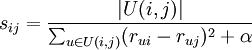where α is a small value that we can play around with.

To build N(u;i), we need a similarity function to determine the similarity of another user v to the given user to u. In other words, we need suv.

The formula for suv is:where α is a small value that we can play around with.

So, we cnow build the set N(u;i) which consists of the k-most similar users that have rated item i (based on maximizing suv) and we can build the set N(i;u) which consists of the k-most similar items that have been rated by user u (based on maximizing sij).

In their article, I am unclear on how α is determined. They say "for some small α".
Determining Interpolation Weights

Next, we need to determine interpolation weights for the prediction.

If using a user-oriented approach, then each weight is wuv.

If using an item-oriented approach, then each weight is wij.

The goal is ultimately to use one of the following formulas for a prediction:or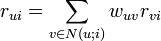If the data is dense enough, then the interpolation can be determined as a least squares problem:

Aw = b

which gives us:

w = A-1b

where A is an invertible K x K matrix defined as:and b ∈ RK where: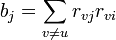Since, it is unreasonable to assume that the data is dense enough, one improvement would be to average each sum over its total number of members. In this case: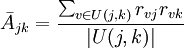and:where U(j,k) is the set of users who rated both item j and item k and U(i,j) is the set of users hwo rated both item i and item j.

But this can be quite inaccurate for very small sets of U(i,j). To remedy this, the article recommends using a baseline value which they avg.

We will create two estimates of the above values:andIn the above estimates, "the parameter β controls the extent of the shrinkage. " For example, in the article, they talk about their reasons for using a β = 500 when used with normalized data.

The value of avg is computed based on the following matrix:where if jk above is a diagonal entry, then avg = the average of diagonal entries of A

and if jk above is a nondiagonal entry, then avg = the average of nondiagonal entries of A

This then completes the formulas as presented in their article on the Neighborhood Based Approach. In my next blog, I will look into code for implementing the kNN approach and see if I am able to reproduce the RMSE scores reported by the BellKor team.

References